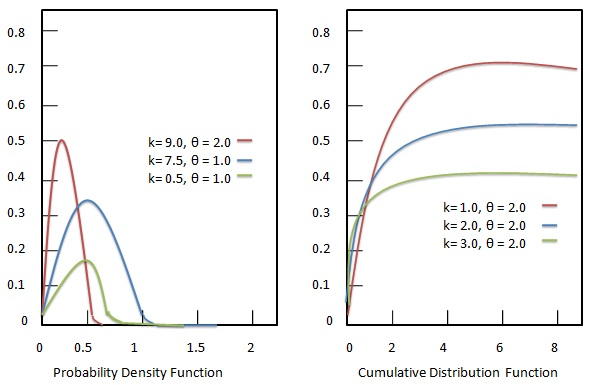# Statistics - Gamma Distribution

#### Class 11th Statistics for Economics

40 Lectures 3.5 hours

#### Statistics

40 Lectures 2 hours

#### An Introduction to Wait Statistics in SQL Server

22 Lectures 1 hours

The gamma distribution represents continuous probability distributions of two-parameter family. Gamma distributions are devised with generally three kind of parameter combinations.

• A shape parameter $k$ and a scale parameter $\theta$.

• A shape parameter $\alpha = k$ and an inverse scale parameter $\beta = \frac{1}{ \theta}$, called as rate parameter.

• A shape parameter $k$ and a mean parameter $\mu = \frac{k}{\beta}$.Each parameter is a positive real numbers. The gamma distribution is the maximum entropy probability distribution driven by following criteria.

## Formula

${E[X] = k \theta = \frac{\alpha}{\beta} \gt 0 \ and \ is \ fixed. \\[7pt] E[ln(X)] = \psi (k) + ln( \theta) = \psi( \alpha) - ln( \beta) \ and \ is \ fixed. }$

Where −

• ${X}$ = Random variable.

• ${\psi}$ = digamma function.

## Characterization using shape $\alpha$ and rate $\beta$

### Probability density function

Probability density function of Gamma distribution is given as:

## Formula

${ f(x; \alpha, \beta) = \frac{\beta^\alpha x^{\alpha - 1 } e^{-x \beta}}{\Gamma(\alpha)} \ where \ x \ge 0 \ and \ \alpha, \beta \gt 0 }$

Where −

• ${\alpha}$ = location parameter.

• ${\beta}$ = scale parameter.

• ${x}$ = random variable.

### Cumulative distribution function

Cumulative distribution function of Gamma distribution is given as:

## Formula

${ F(x; \alpha, \beta) = \int_0^x f(u; \alpha, \beta) du = \frac{\gamma(\alpha, \beta x)}{\Gamma(\alpha)}}$

Where −

• ${\alpha}$ = location parameter.

• ${\beta}$ = scale parameter.

• ${x}$ = random variable.

• ${\gamma(\alpha, \beta x)}$ = lower incomplete gamma function.

## Characterization using shape $k$ and scale $\theta$

### Probability density function

Probability density function of Gamma distribution is given as:

## Formula

${ f(x; k, \theta) = \frac{x^{k - 1 } e^{-\frac{x}{\theta}}}{\theta^k \Gamma(k)} \ where \ x \gt 0 \ and \ k, \theta \gt 0 }$

Where −

• ${k}$ = shape parameter.

• ${\theta}$ = scale parameter.

• ${x}$ = random variable.

• ${\Gamma(k)}$ = gamma function evaluated at k.

### Cumulative distribution function

Cumulative distribution function of Gamma distribution is given as:

## Formula

${ F(x; k, \theta) = \int_0^x f(u; k, \theta) du = \frac{\gamma(k, \frac{x}{\theta})}{\Gamma(k)}}$

Where −

• ${k}$ = shape parameter.

• ${\theta}$ = scale parameter.

• ${x}$ = random variable.

• ${\gamma(k, \frac{x}{\theta})}$ = lower incomplete gamma function.